Precalculus : Evaluate Expressions That Include the Inverse Tangent, Cosecant, Secant, or Cotangent Function

Example Questions

Example Question #1 : Evaluate Expressions That Include The Inverse Tangent, Cosecant, Secant, Or Cotangent Function

Approximate: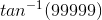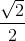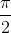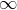Explanation::

There is a restriction for the range of the inverse tangent function from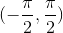.

The inverse tangent of a value asks for the angle where the coordinate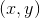lies on the unit circle under the condition that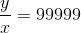.  For this to be valid on the unit circle, themust be very close to 1, with anvalue also very close to zero, but cannot equal to zero sincewould be undefined.

The point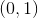is located on the unit circle when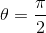, but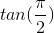is invalid due to the existent asymptote at this angle.

An example of a point very close tothat will yieldcan be written as: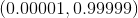Therefore, the approximated rounded value ofis.

Example Question #32 : Graphs And Inverses Of Trigonometric Functions

Evaluate: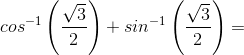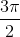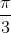Explanation:

To determine the value of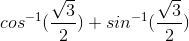, solve each of the terms first.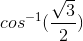The inverse cosine has a domain and range restriction.

The domain exists from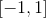, and the range from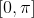.  The inverse cosine asks for the angle when the x-value of the existing coordinate is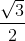.  The only possibility is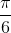since the coordinate can only exist in the first quadrant.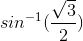The inverse sine also has a domain and range restriction.

The domain exists from, and the range from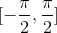.  The inverse sine asks for the angle when the y-value of the existing coordinate is.  The only possibility issince the coordinate can only exist in the first quadrant.

Therefore: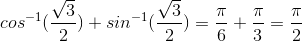Example Question #33 : Graphs And Inverses Of Trigonometric Functions

Determine the correct value of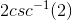in degrees.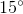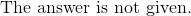Explanation:

Rewrite and evaluate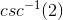.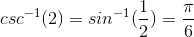The inverse sine of one-half issinceis the y-value of the coordinate when the angle is.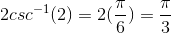To convert from radians to degrees, replacewith 180.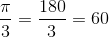Example Question #34 : Graphs And Inverses Of Trigonometric Functions

Evaluate the following: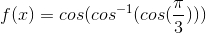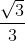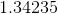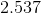Explanation:

For this particular problem we need to recall that the inverse cosine cancels out the cosine therefore,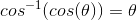.

So the expression just becomes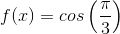From here, recall the unit circle for specific angles such as.

Thus,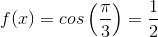.

All Precalculus Resources